sk|cz|

# Logarithmic Equations

1. Solve: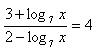x > 0
Solution:
3+log7x = 8 – 4log7x
5log7x  = 5
log7x = 1
x = 71 = 7

K = {7}

2. Solve: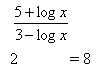x > 0
Solution: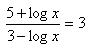5+logx = 9-3logx
4logx  = 4
logx  = 1
x = 101= 10

K = {10}

3.Solve: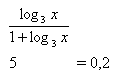x > 0
Solution: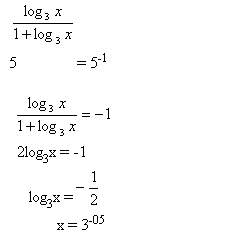K = {3-0,5}

4. Solve:

log3(5+4.log2(x-1)) = 2                 x > 1
Solution:
log3(5+4.log2(x-1)) = 2
log3(5+4.log2(x-1)) = log39
5+4.log2(x-1) = 9
4.log2(x-1) = 4
log2(x-1) = 1
x-1 = 21
x = 3

K = {3}

5. Solve:

log(x+5) - log(x-1) = 1-log2                 x > 1
Solution: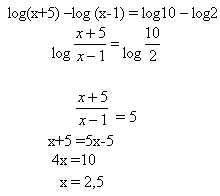K = {2,5}

6. Solve:

log(x+2) + log(x-7) = 2.log(x-4)               x > 7
Solution:
log(x+2) + log(x-7) = 2.log(x-4)
log (x+2)(x-7) = log(x-4)2
(x+2)(x-7) = (x-4)2
x2-5x-14 = x2-8x +16
3x = 30
x = 10

K = {10}

7. Solve:

log5x +log (2x + 3) = 1 + 2.log(3-x)                x < 3
Solution:
log5x +log (2x + 3) = 1 + 2.log(3-x)
log5x + log(2x + 3) = log10 + log(3-x)2
log(5x.(2x +3)) = log (10.(3-x)2)
5x.(2x +3) = 10.(3-x)2
10x2 +15x = 10.(9-6x + x2)
10x2 + 15x = 90-60x +10x2
75x = 90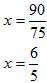8. Solve:

log(1+x)–log(1-x) = log(x+3)-log(4-x)            x < 1
Solution: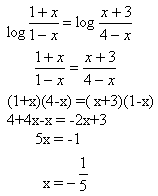9. Solve:

2log3x2 + 3log4x3 = 4log2x2 +4log6x                    x > 0
Solution:
2log3x2 + 3log4x3 = 4log2x2 +4log6x
log9x4 + log64x9 = log16x8 + log1296x4
log(576x13) = log(20736x12)
576x13 = 20736x12/:576x12
x = 36

K = {36}

10.

Solve in real numbers: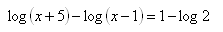Solution: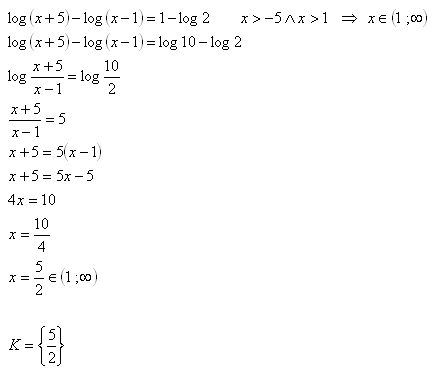11.Solve in real numbers: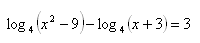Solution: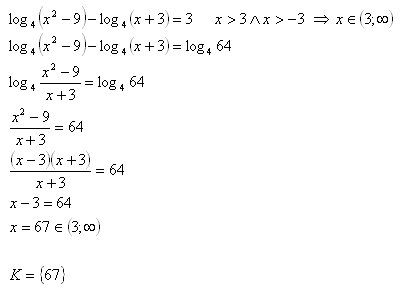12.Solve in real numbers: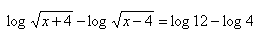Solution:13.Solve in real numbers: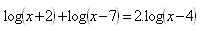Solution: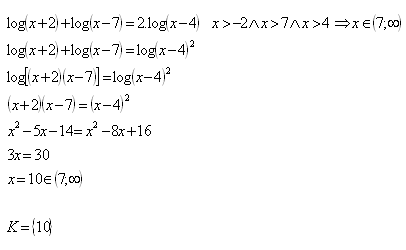14.Solve in real numbers: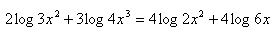Solution: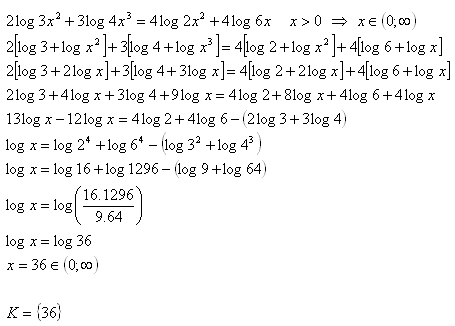15.Solve in real numbers: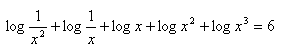Solution: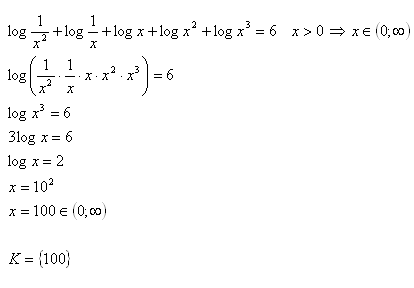16.Solve in real numbers: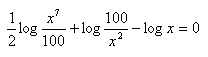Solution: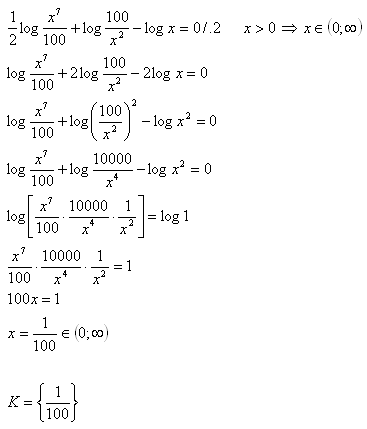17.Solve in real numbers: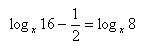Solution: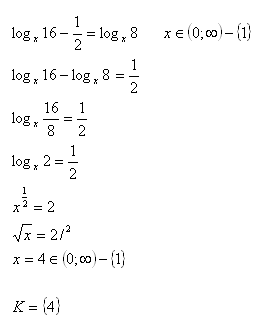18.Solve in real numbers: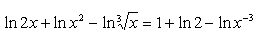Solution: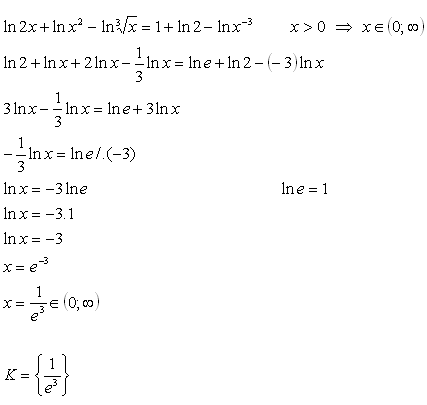19.Solve in real numbers: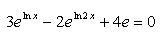Solution: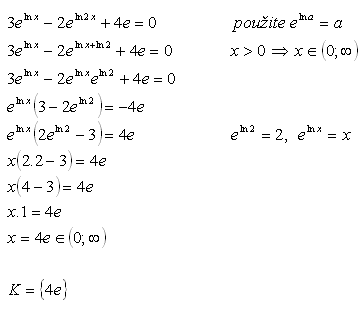20.Solve in real numbers: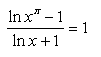Solution: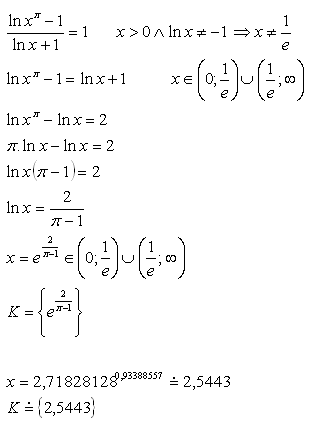Logarithmic equations – further exercise: